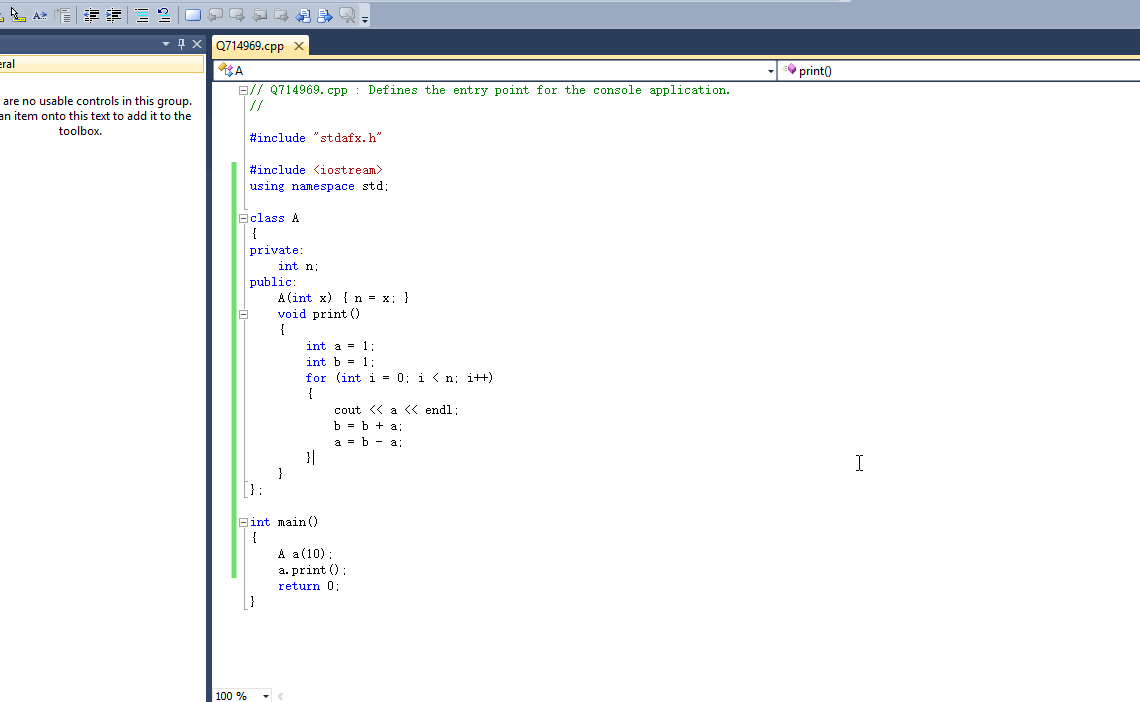2018-11-28 05:25

# 这个题目啥意思，看不懂

,f2=1,f3=2,fn=fn-1+fn+1

• 点赞
• 写回答
• 关注问题
• 收藏
• 邀请回答

#### 2条回答默认 最新

•blownewbee 2018-11-28 06:00
已采纳

# 如果问题得到解决，请点我回答左上角的采纳和向上的箭头，谢谢

``````#include <iostream>
using namespace std;

class A
{
private:
int n;
public:
A(int x) { n = x; }
void print()
{
int a = 1;
int b = 1;
for (int i = 0; i < n; i++)
{
cout << a << endl;
b = b + a;
a = b - a;
}
}
};

int main()
{
A a(10);
a.print();
return 0;
}
``````点赞 评论
•鱼在沙漠 2018-11-28 05:57
``````#include <stdio.h>
#include <vector>
using std::vector;
class Fibonacci{
public:
Fibonacci(int cnt):n(cnt){}
void print(){
if (n <= 2)
{
if(n == 1){
printf("1\n");
}
else{
printf("1 1\n");
}
}
arr.push_back(1);
arr.push_back(1);
int a = 1;
int b = 1;
int c = 1;
for (int i = 3; i <= n; i++)
{
a = b;
b = c;
c = a + b;
arr.push_back(c);
}
for(int i = 0; i < arr.size(); i++){
printf("%d ", arr[i]);
}
printf("\n");
}
private:
vector<int> arr;
int n;
};
int main(){
Fibonacci fibon(6);
fibon.print();
return 0;
}

``````
点赞 评论